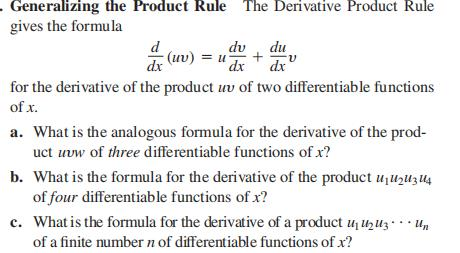### Still have math questions?

Trigonometry
QuestionGeneralizing the Product Rule The Derivative Product Rule gives the formula $$\frac { d } { d x } ( u v ) = u \frac { d v } { d x } + \frac { d u } { d x } v$$ for the derivative of the product $$u v$$ of two differentiable functions of $$x$$ .

a. What is the analogous formula for the derivative of the prod- uct $$u v w$$ of three differentiable functions of $$x$$ ?

b. What is the formula for the derivative of the product $$u _ { 1 } u _ { 2 } u _ { 3 } u _ { 4 }$$ of four differentiable functions of $$x$$ ?

$$\left. \begin{array} { l } { \text { (a) } \frac { d } { d x } ( u v w ) = u v w ^ { \prime } + u v ^ { \prime } w + u ^ { \prime } v w } \\ { \text { (b) } \frac { d } { d x } ( u _ { 1 } u _ { 2 } u _ { 3 } u _ { 4 } ) = u _ { 1 } u _ { 2 } u _ { 3 } u _ { 4 } ^ { \prime } + u _ { 1 } u _ { 2 } u _ { 3 } ^ { \prime } u _ { 4 } + u _ { 1 } u _ { 2 } ^ { \prime } u _ { 3 } u _ { 4 } + } \\ { u _ { 1 } ^ { \prime } u _ { 2 } u _ { 3 } u _ { 4 } } \\ { \text { (c) } \frac { d } { d x } ( u _ { 1 } \cdots u _ { n } ) = u _ { 1 } u _ { 2 } \cdots u _ { n - 1 } u _ { n } ^ { \prime } + u _ { 1 } u _ { 2 } \cdots u _ { n - 2 } u _ { n - 1 } ^ { \prime } u _ { n } + } \\ { \cdots + u _ { 1 } ^ { \prime } u _ { 2 } \cdots u _ { n } } \end{array} \right.$$